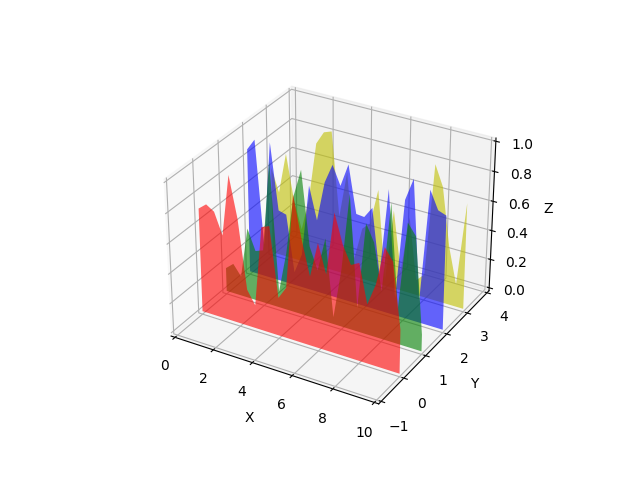# Generate polygons to fill under 3D line graph¶

Demonstrate how to create polygons which fill the space under a line graph. In this example polygons are semi-transparent, creating a sort of 'jagged stained glass' effect.from matplotlib.collections import PolyCollection
import matplotlib.pyplot as plt
import numpy as np

# Fixing random state for reproducibility
np.random.seed(19680801)

def polygon_under_graph(xlist, ylist):
"""
Construct the vertex list which defines the polygon filling the space under
the (xlist, ylist) line graph.  Assumes the xs are in ascending order.
"""
return [(xlist, 0.), *zip(xlist, ylist), (xlist[-1], 0.)]

# Make verts a list such that verts[i] is a list of (x, y) pairs defining
# polygon i.
verts = []

# Set up the x sequence
xs = np.linspace(0., 10., 26)

# The ith polygon will appear on the plane y = zs[i]
zs = range(4)

for i in zs:
ys = np.random.rand(len(xs))
verts.append(polygon_under_graph(xs, ys))

poly = PolyCollection(verts, facecolors=['r', 'g', 'b', 'y'], alpha=.6)